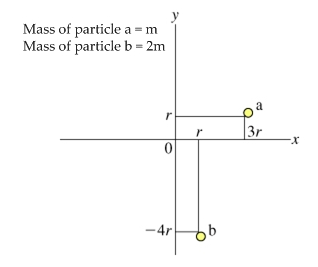# Problem: Find the moment of inertia Ix of particle a with respect to the x axis (that is, if the x axis is the axis of rotation), the moment of inertia Iy of particle a with respect to the y axis, and the moment of inertia Iz of particle a with respect to the z axis (the axis that passes through the origin perpendicular to both the xand y axes)Express your answers in terms of m and r separated by commas.

###### FREE Expert Solution

Moment of inertia of a point mass:

$\overline{){\mathbf{I}}{\mathbf{=}}{\mathbf{M}}{{\mathbf{R}}}^{{\mathbf{2}}}}$

The perpendicular distance of particle a from the x-axis is r.###### Problem Details

Find the moment of inertia Ix of particle a with respect to the x axis (that is, if the x axis is the axis of rotation), the moment of inertia Iy of particle a with respect to the y axis, and the moment of inertia Iz of particle a with respect to the z axis (the axis that passes through the origin perpendicular to both the xand y axes)

Express your answers in terms of m and r separated by commas.